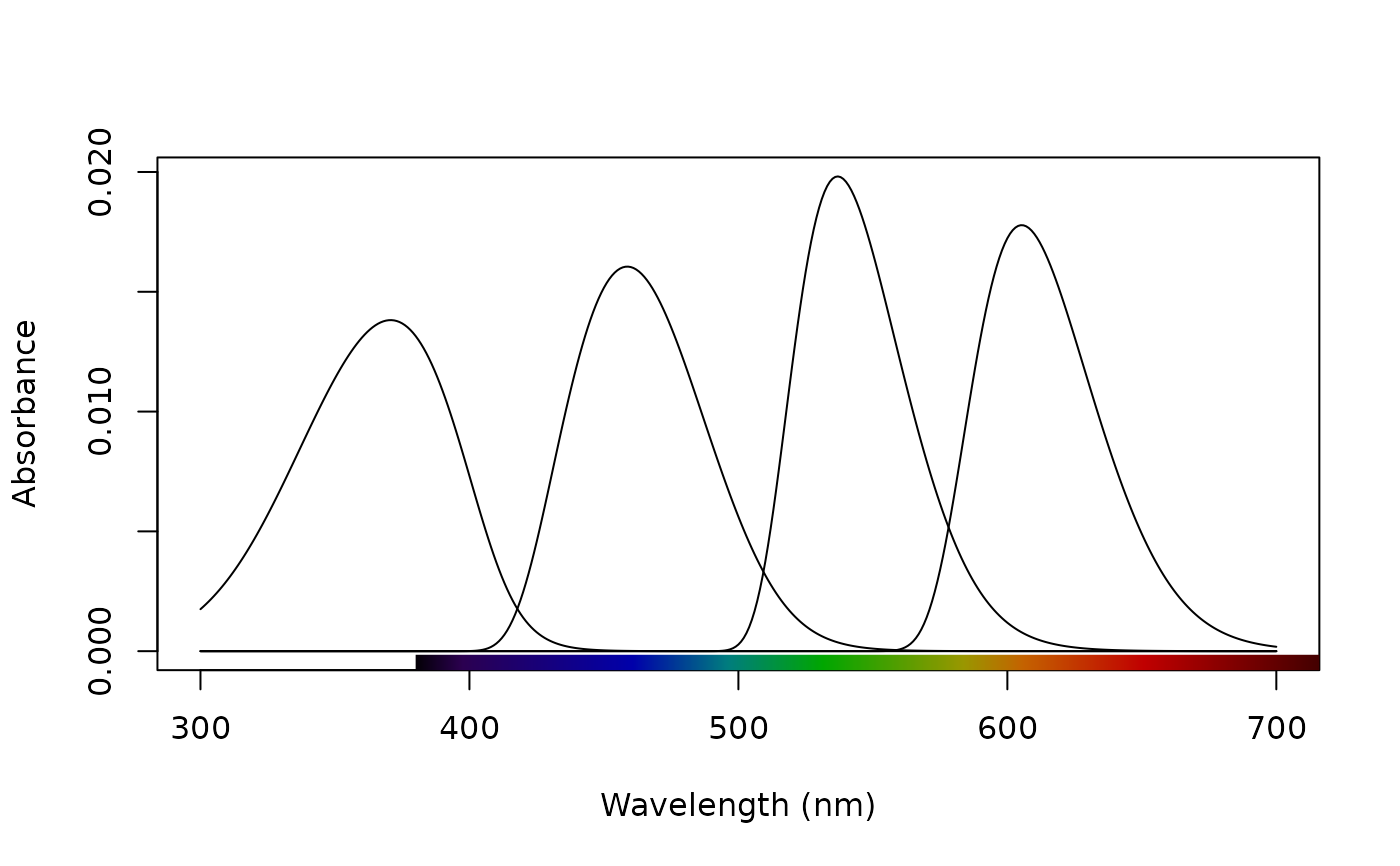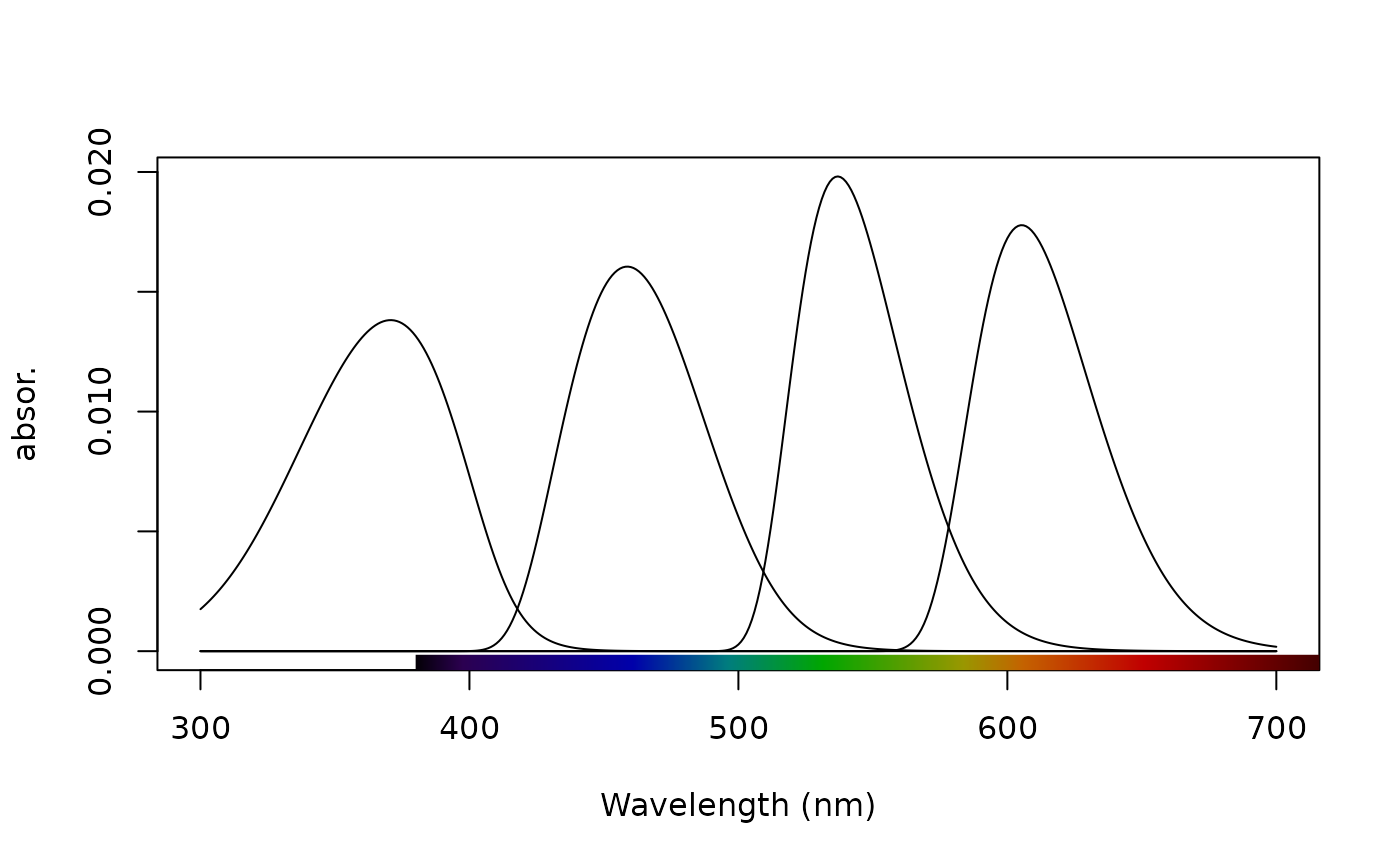Plot absorbance spectra from sensmodel()

# S3 method for sensmod
plot(
x,
select = NULL,
type = c("overlay", "stack", "heatmap"),
varying = NULL,
n = 100,
labels = FALSE,
labels.stack = NULL,
labels.cex = 1,
wl.guide = TRUE,
...
)

## Arguments

x

(required) a data frame, possibly an object of class rspec, with a column with wavelength data, named 'wl', and the remaining column containing spectra to plot.

select

specification of which spectra to plot. Can be a numeric vector or factor (e.g., sex == "male")

type

what type of plot should be drawn. Possibilities are:

• overlay (default) for plotting multiple spectra in a single panel with a common y-axis.

• stack for plotting multiple spectra in a vertical arrangement.

• heatmap for plotting reflectance values by wavelength and a third variable (varying).

varying

a numeric vector giving values for y-axis in type = "heatmap".

n

number of bins with which to interpolate colors and varying for the heatplot.

labels

logical. Add labels identifying each spectrum to the outer plot margin? Defaults to FALSE. Ignored when type = 'heatmap'.

labels.stack

a vector of labels for spectra when labels = TRUE. Defaults to the column names from spectral data. Note you will likely want to adjust the plot margins to accommodate the text labels. See ?par() for guidance on setting margins.

labels.cex

size of the text labels when labels = TRUE.

wl.guide

logical determining whether visible light spectrum should be added to the x-axis.

...

additional arguments passed to plot() (or image() for "heatmap").

plot.rspec(), sensmodel()

## Examples

# Blue tit visual system based on Hart et al (2000)
bluesens <- sensmodel(c(371, 448, 502, 563),
beta = FALSE,
lambdacut = c(330, 413, 507, 572),
oiltype = c("T", "C", "Y", "R"), om = TRUE
)
plot(bluesens)# Alternatively, you can specify your own ylab
plot(bluesens, ylab = "absor.")